# 多元线性回归模型

多元线性回归是一元线性回归的多维运用。在一元线性回归中，我们用自变量系数表示对自变量与因变量之间的解释因素。那么，在多元线性回归中，我们引入多个解释变量，对同一个个体在不同方面的解释进行多维度的衡量。

为了便于理解，我们可以假设有一幢楼进行售卖，用y表示房子的房价，x表示这间房子的不同解释因素，如：面积、位置、采光度、装修等等（影响一间房子的价格绝不仅仅是面积的大小，因此这样的解释是合理的）。

那么，我们就要列举出一个能够综合评判房屋价格的一个数学模型，通过不同维度的计量，从而得到一个能够较全面衡量一间房屋综合价值的数值（类似于国民GDP）。

由此，我们便引入了多元线性回归模型，为了便于观察，我们还引入了基于矩阵运算的模型分析。

通常情况下，一般的多元回归模型写法很多，例如：这样写太过于繁杂，并且在实际求解的过程中并不能简化我们的运算。为此，引入矩阵运算。

假定x的矩阵为：X=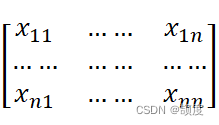，对应的θ的矩阵为：θ=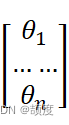在X中添加一个列向量1，得到：X=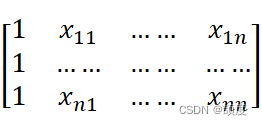通过矩阵运算，可以将hθ（x）=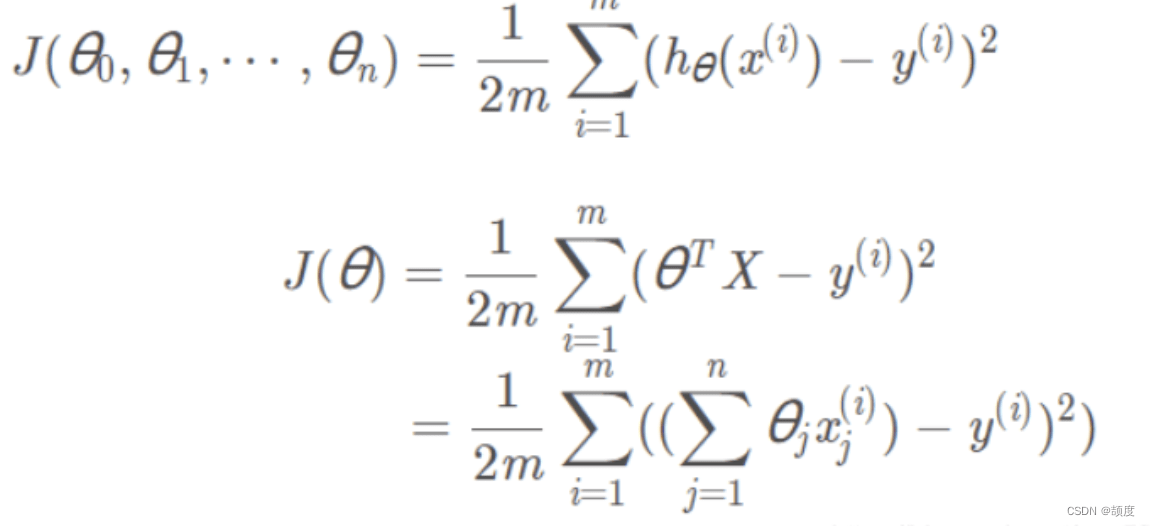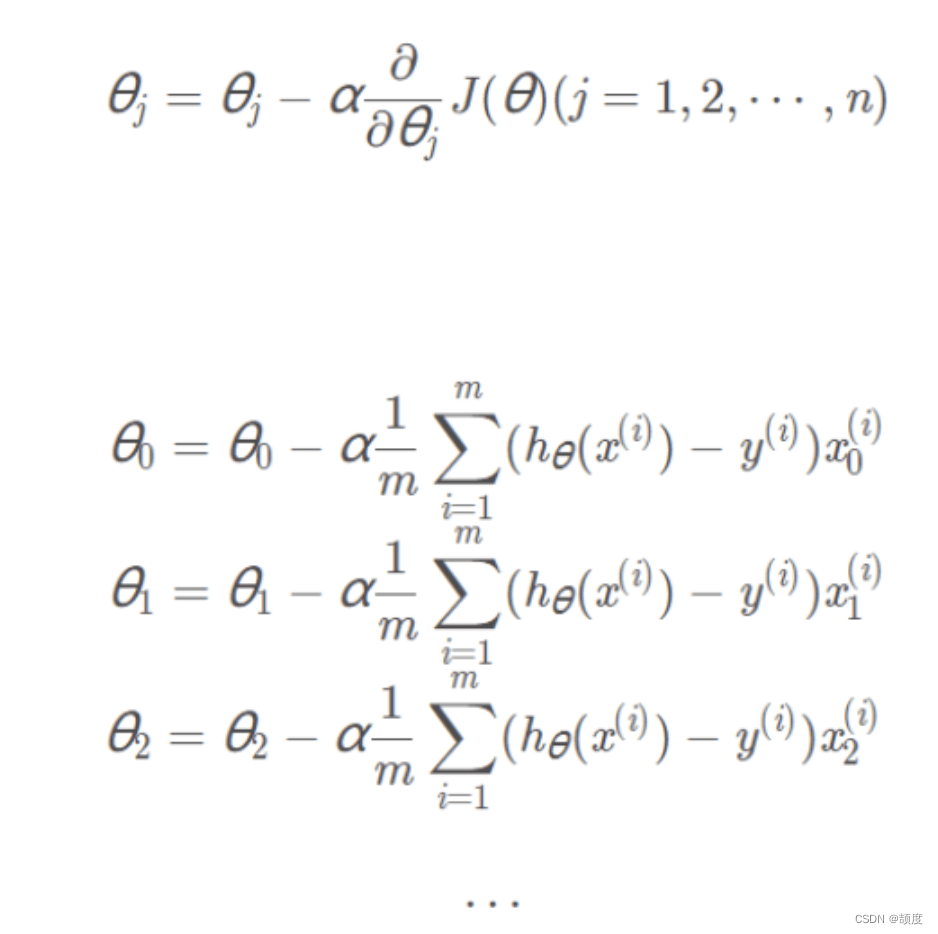Iris 鸢尾花数据集内包含 3 类，分别为山鸢尾（Iris-setosa）、变色鸢尾（Iris-versicolor）和维吉尼亚鸢尾（Iris-virginica），共 150 条记录，每类各 50 个数据，每条记录都有 4 项特征：花萼长度、花萼宽度、花瓣长度、花瓣宽度，可以通过这 4 个特征预测鸢尾花卉属于哪一品种。 这是本文章所使用的鸢尾花数据集： sl：花萼长度 ；sw：花萼宽度 ；pl：花瓣长度 ；pw：花瓣宽度； type：类别：（Iris-setosa、Iris-versicolor、Iris-virginica 三类）

部分展示：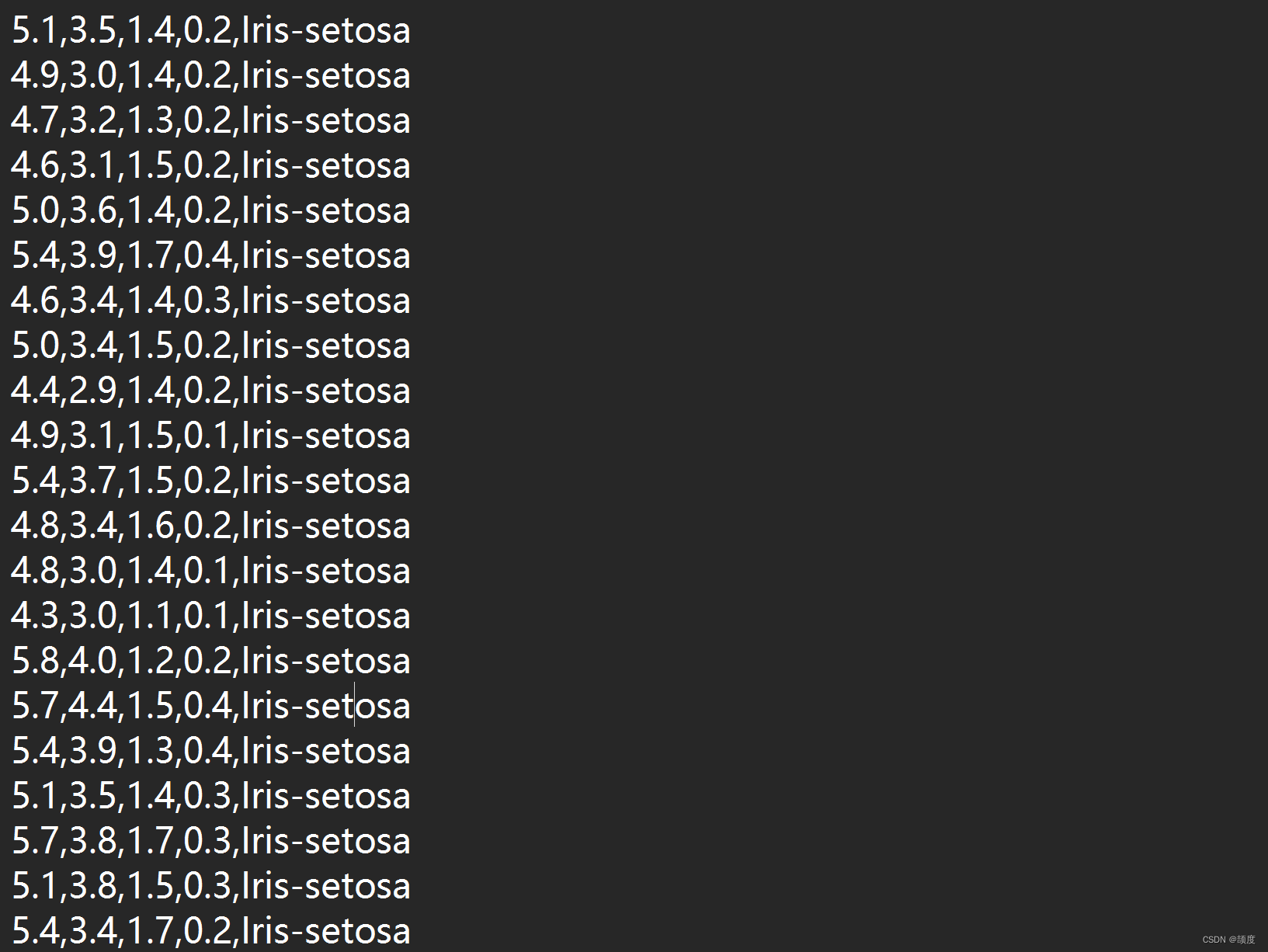``````import pandas as pd
import numpy as np
import time
import random
def MGD_train(X, y, alpha=0.0001, maxIter=1000, theta_old=None):
'''
MGD训练线性回归
传入:
X       :  已知数据
y       :  标签
alpha   :  学习率
maxIter :  总迭代次数
返回:
theta : 权重参数
'''
# 初始化权重参数
theta = np.ones(shape=(X.shape,))
if not theta_old is None:
# 假装是断点续训练
theta = theta_old.copy()

# axis=1 表示横轴，方向从左到右；axis=0 表示纵轴，方向从上到下
for i in range(maxIter):
# 预测
y_pred = np.sum(X * theta, axis=1)
# 全部数据得到的梯度
gradient = np.average((y - y_pred).reshape(-1, 1) * X, axis=0)
# 更新学习率
return theta

def SGD_train(X, y, alpha=0.0001, maxIter=1000, theta_old=None):
'''
SGD训练线性回归
传入:
X       :  已知数据
y       :  标签
alpha   :  学习率
maxIter :  总迭代次数

返回:
theta : 权重参数
'''
# 初始化权重参数
theta = np.ones(shape=(X.shape,))
if not theta_old is None:
# 假装是断点续训练
theta = theta_old.copy()
# 数据数量
data_length = X.shape
for i in range(maxIter):
# 随机选择一个数据
index = np.random.randint(0, data_length)
# 预测
y_pred = np.sum(X[index, :] * theta)
# 一条数据得到的梯度
gradient = (y[index] - y_pred) * X[index, :]
# 更新学习率
return theta

def MBGD_train(X, y, alpha=0.0001, maxIter=1000, batch_size=10, theta_old=None):
'''
MBGD训练线性回归
传入:
X          :  已知数据
y          :  标签
alpha      :  学习率
maxIter    :  总迭代次数
batch_size :  没一轮喂入的数据数``````
``````
返回:
theta : 权重参数
'''
# 初始化权重参数
theta = np.ones(shape=(X.shape,))

if not theta_old is None:
# 假装是断点续训练
theta = theta_old.copy()

# 所有数据的集合
all_data = np.concatenate([X, y.reshape(-1, 1)], axis=1)
for i in range(maxIter):
# 从全部数据里选 batch_size 个 item
X_batch_size = np.array(random.choices(all_data, k=batch_size))

# 重新给 X, y 赋值
X_new = X_batch_size[:, :-1]
y_new = X_batch_size[:, -1]

# 将数据喂入，更新 theta
theta = MGD_train(X_new, y_new, alpha=0.0001, maxIter=1, theta_old=theta)
return theta

def GD_predict(X, theta):
'''
用于预测的函数
传入:
X     : 数据
theta : 权重
返回:
y_pred: 预测向量
'''
y_pred = np.sum(theta * X, axis=1)
# 实数域空间 -> 离散三值空间，则需要四舍五入
y_pred = (y_pred + 0.5).astype(int)
return y_pred

def calc_accuracy(y, y_pred):
'''
计算准确率
传入:
y        : 标签
y_pred   : 预测值
返回:
accuracy : 准确率
'''
return np.average(y == y_pred) * 100
# 读取数据
iris_raw_data = pd.read_csv('iris.data', names  =['sepal length', 'sepal width', 'petal length', 'petal width', 'class'])
# 将三种类型映射成整数
Iris_dir = {'Iris-setosa': 1, 'Iris-versicolor': 2, 'Iris-virginica': 3}
iris_raw_data['class'] = iris_raw_data['class'].apply(lambda x:Iris_dir[x])
# 训练数据 X
iris_data = iris_raw_data.values[:, :-1]
# 标签 y
y = iris_raw_data.values[:, -1]
# 用 MGD 训练的参数
start = time.time()
theta_MGD = MGD_train(iris_data, y)
run_time = time.time() - start
y_pred_MGD = GD_predict(iris_data, theta_MGD)
print("MGD训练1000轮得到的准确率{:.2f}% 运行时间是{:.2f}s".format(calc_accuracy(y, y_pred_MGD), run_time))
# 用 SGD 训练的参数
start = time.time()
theta_SGD = SGD_train(iris_data, y)
run_time = time.time() - start
y_pred_SGD = GD_predict(iris_data, theta_SGD)
print("SGD训练1000轮得到的准确率{:.2f}% 运行时间是{:.2f}s".format(calc_accuracy(y, y_pred_SGD), run_time))
# 用 MBGD 训练的参数
start = time.time()
theta_MBGD = MBGD_train(iris_data, y)
run_time = time.time() - start
y_pred_MBGD = GD_predict(iris_data, theta_MBGD)
print("MBGD训练1000轮得到的准确率{:.2f}% 运行时间是{:.2f}s".format(calc_accuracy(y, y_pred_MBGD), run_time))``````